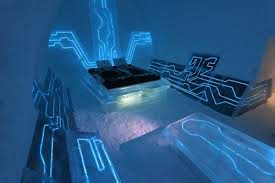Published on Sep 16, 2019

### Abstract

The main aim of this project is to calculate and display the traveling amount, traveled distance in kilo meter, total time of travel, waiting time of the vehicles etc.

### PROJECT DESCRIPTION:

This project is used to calculate the important parameters of a traveling vehicle and to calculate the travel fare using these data. Nowadays call taxi like vehicles are used often and in these if we implement this fare calculating method, then it will be very useful for the public to pay accurately.

Here tacho meter like sensor is placed across the wheel to detect the RPM and this value is given to the micro controller and with the help of this the distance traveled can be calculated accurately.

When the passenger enters/leaves the vehicle the driver has to press a switch so that the timer in the controller gets turned on/off and it is used to calculate the total time of travel. Also he has to press another switch to calculate the waiting time and this time is taken to charge the passenger.

These parameters are detected using appropriate methods and the travel fare is calculated accurately using micro controller and it is displayed by means of LCD

### MATERIALS USED:

• Micro controller 89c51

• 2 line LCD

• Power supply

• sensors

• keys